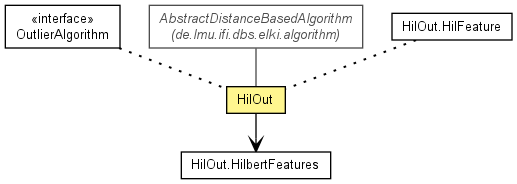de.lmu.ifi.dbs.elki.algorithm.outlier

## Class HilOut<O extends NumberVector<O,?>>• Type Parameters:
`O` - Object type
All Implemented Interfaces:
Algorithm, OutlierAlgorithm, InspectionUtilFrequentlyScanned, Parameterizable

```@Title(value="Fast Outlier Detection in High Dimensional Spaces")
@Description(value="Algorithm to compute outliers using Hilbert space filling curves")
@Reference(authors="F. Angiulli, C. Pizzuti",
title="Fast Outlier Detection in High Dimensional Spaces",
booktitle="Proc. European Conference on Principles of Knowledge Discovery and Data Mining (PKDD\'02)",
url="http://dx.doi.org/10.1145/375663.375668")
public class HilOut<O extends NumberVector<O,?>>
extends AbstractDistanceBasedAlgorithm<O,DoubleDistance,OutlierResult>
implements OutlierAlgorithm```
Fast Outlier Detection in High Dimensional Spaces Outlier Detection using Hilbert space filling curves Reference:

F. Angiulli, C. Pizzuti:
Fast Outlier Detection in High Dimensional Spaces.
In: Proc. European Conference on Principles of Knowledge Discovery and Data Mining (PKDD'02), Helsinki, Finland, 2002.

• ### Nested Class Summary

Nested Classes
Modifier and Type Class and Description
`(package private) class ` `HilOut.HilbertFeatures`
Class organizing the data points along a hilbert curve.
`(package private) static class ` `HilOut.HilFeature`
Hilbert representation of a single object.
`static class ` `HilOut.Parameterizer<O extends NumberVector<O,?>>`
Parameterization class
`static class ` `HilOut.ScoreType`
Type of output: all scores (upper bounds) or top n only
• ### Field Summary

Fields
Modifier and Type Field and Description
`private int` `capital_n`
Set sizes, total and current iteration
`private int` `capital_n_star`
Set sizes, total and current iteration
`private int` `d`
Set sizes, total and current iteration
`private DistanceQuery<O,DoubleDistance>` `distq`
Distance query
`private int` `h`
Hilbert precision
`private int` `k`
Number of nearest neighbors
`private static Logging` `logger`
The logger for this class.
`private int` `n`
Number of outliers to compute exactly
`private int` `n_star`
Set sizes, total and current iteration
`private double` `omega_star`
Outlier threshold
`private double` `t`
LPNorm p parameter
`private Enum<HilOut.ScoreType>` `tn`
Reporting mode: exact (top n) only, or all
• ### Fields inherited from class de.lmu.ifi.dbs.elki.algorithm.AbstractDistanceBasedAlgorithm

`DISTANCE_FUNCTION_ID`
• ### Constructor Summary

Constructors
Modifier Constructor and Description
`protected ` ```HilOut(LPNormDistanceFunction distfunc, int k, int n, int h, Enum<HilOut.ScoreType> tn)```
Constructor.
• ### Method Summary

Methods
Modifier and Type Method and Description
`TypeInformation[]` `getInputTypeRestriction()`
Get the input type restriction used for negotiating the data query.
`protected Logging` `getLogger()`
Get the (STATIC) logger for this class.
`private void` ```innerScan(HilOut.HilbertFeatures hf, int i, int maxcount)```
innerScan function calculates new upper and lower bounds and inserts the points of the neighborhood the bounds are based on in the NN Set
`OutlierResult` ```run(Database database, Relation<O> relation)```
`private void` ```scan(HilOut.HilbertFeatures hf, int k0)```
Scan function performs a squential scan over the data.
`private void` `trueOutliers(HilOut.HilbertFeatures h)`
trueOutliers function updates n_star
• ### Methods inherited from class de.lmu.ifi.dbs.elki.algorithm.AbstractDistanceBasedAlgorithm

`getDistanceFunction`
• ### Methods inherited from class de.lmu.ifi.dbs.elki.algorithm.AbstractAlgorithm

`makeParameterDistanceFunction, run`
• ### Methods inherited from class java.lang.Object

`clone, equals, finalize, getClass, hashCode, notify, notifyAll, toString, wait, wait, wait`
• ### Methods inherited from interface de.lmu.ifi.dbs.elki.algorithm.outlier.OutlierAlgorithm

`run`
• ### Field Detail

• #### logger

`private static final Logging logger`
The logger for this class.
• #### k

`private int k`
Number of nearest neighbors
• #### n

`private int n`
Number of outliers to compute exactly
• #### h

`private int h`
Hilbert precision
• #### t

`private double t`
LPNorm p parameter
• #### tn

`private Enum<HilOut.ScoreType> tn`
Reporting mode: exact (top n) only, or all
• #### distq

`private DistanceQuery<O extends NumberVector<O,?>,DoubleDistance> distq`
Distance query
• #### capital_n

`private int capital_n`
Set sizes, total and current iteration
• #### n_star

`private int n_star`
Set sizes, total and current iteration
• #### capital_n_star

`private int capital_n_star`
Set sizes, total and current iteration
• #### d

`private int d`
Set sizes, total and current iteration
• #### omega_star

`private double omega_star`
Outlier threshold
• ### Constructor Detail

• #### HilOut

```protected HilOut(LPNormDistanceFunction distfunc,
int k,
int n,
int h,
Enum<HilOut.ScoreType> tn)```
Constructor.
Parameters:
`k` - Number of Next Neighbors
`n` - Number of Outlier
`h` - Number of Bits for precision to use - max 32
`tn` - TopN or All Outlier Rank to return
• ### Method Detail

• #### run

```public OutlierResult run(Database database,
Relation<O> relation)```
• #### scan

```private void scan(HilOut.HilbertFeatures hf,
int k0)```
Scan function performs a squential scan over the data.
Parameters:
`hf` - the hilbert features
`k0` -
• #### innerScan

```private void innerScan(HilOut.HilbertFeatures hf,
int i,
int maxcount)```
innerScan function calculates new upper and lower bounds and inserts the points of the neighborhood the bounds are based on in the NN Set
Parameters:
`i` - position in pf of the feature for which the bounds should be calculated
`maxcount` - maximal size of the neighborhood
• #### trueOutliers

`private void trueOutliers(HilOut.HilbertFeatures h)`
trueOutliers function updates n_star
Parameters:
`h` - the HilberFeatures
• #### getLogger

`protected Logging getLogger()`
Description copied from class: `AbstractAlgorithm`
Get the (STATIC) logger for this class.
Specified by:
`getLogger` in class `AbstractAlgorithm<OutlierResult>`
Returns:
the static logger
• #### getInputTypeRestriction

`public TypeInformation[] getInputTypeRestriction()`
Description copied from class: `AbstractAlgorithm`
Get the input type restriction used for negotiating the data query.
Specified by:
`getInputTypeRestriction` in interface `Algorithm`
Specified by:
`getInputTypeRestriction` in class `AbstractAlgorithm<OutlierResult>`
Returns:
Type restriction
 Release 0.5.0 (2012-07-02_1155)